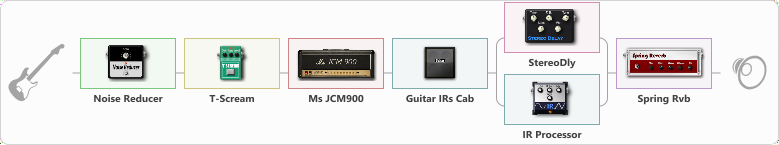# Heavy Jcm900

Discussion in 'ToneLib-GFX presets' started by truss van halen, Dec 6, 2020.

1. ### truss van halenWell-Known Member

Heavy Jcm900

Preset name: My Heavy JCM900 (2)

Effects chain:Effect: "Noise Reducer" (Dynamics / Filter), active - "yes"
{
"Sens" = 100
"Mode" = Hard
}

Effect: "T-Scream" (Overdrive / Distortion), active - "yes"
{
"Drive" = 0
"Tone" = 63
"Level" = 100
}

Effect: "Ms JCM900" (Amp simulators), active - "yes"
{
"Gain" = 50
"Bass" = 35
"Middle" = 63
"Treble" = 60
"Presence" = 50
"Master" = 50
"Level (dB)" = 0
}

Effect: "Guitar IRs Cab" (Cabinets), active - "yes"
{
"Model" = Fender Deluxe Rev (1x12")
"Mic Position" = Middle
"Mic Distance" = Near
"Low Cut (Hz)" = 60
"Hi Cut (kHz)" = 20.0
"Mix" = 100
"Level (dB)" = 0
}

Effect: "Splitter" (Dynamics / Filter), active - "yes"
{
"A-Bypass" = Off
"A-Pan" = 0
"A-Level" = 55
"B-Bypass" = Off
"B-Pan" = 0
"B-Level" = 55

'A' branch:
{

Effect: "StereoDly" (Delay), active - "yes"
{
"Time" = 836
"Feedback" = 38
"Tone" = 76
"Sens" = 0
"Mix" = 52
}
}
'B' branch:
{

Effect: "IR Processor" (Cabinets), active - "yes"
{
"IR" = Cab IR Sample3
"Low Cut (Hz)" = 0
"Hi Cut (kHz)" = 20.0
"Mix" = 100
"Level (dB)" = 0
}
}
}

Effect: "Spring Rvb" (Reverberation), active - "yes"
{
"Time" = 5.4
"PreDelay" = 0
"LoDamp" = 16
"HiDamp" = 20
"Mix" = 42
}

Note: You will need to download and install the ToneLib-GFX software to use the preset.

File size:
2.5 KB
Views:
2,393